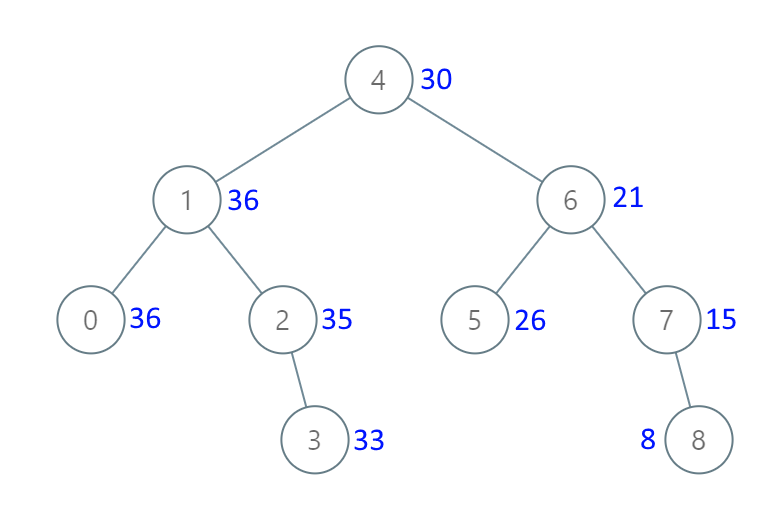## 1038. Binary Search Tree to Greater Sum Tree

Given the `root` of a Binary Search Tree (BST), convert it to a Greater Tree such that every key of the original BST is changed to the original key plus sum of all keys greater than the original key in BST.

As a reminder, a binary search tree is a tree that satisfies these constraints:

• The left subtree of a node contains only nodes with keys less than the node's key.
• The right subtree of a node contains only nodes with keys greater than the node's key.
• Both the left and right subtrees must also be binary search trees.

Note: This question is the same as 538: https://leetcode.com/problems/convert-bst-to-greater-tree/

Example 1:```Input: root = [4,1,6,0,2,5,7,null,null,null,3,null,null,null,8]
Output: [30,36,21,36,35,26,15,null,null,null,33,null,null,null,8]
```

Example 2:

```Input: root = [0,null,1]
Output: [1,null,1]
```

Example 3:

```Input: root = [1,0,2]
Output: [3,3,2]
```

Example 4:

```Input: root = [3,2,4,1]
Output: [7,9,4,10]
```

Constraints:

• The number of nodes in the tree is in the range `[1, 100]`.
• `0 <= Node.val <= 100`
• All the values in the tree are unique.
• `root` is guaranteed to be a valid binary search tree.

## Rust Solution

``````struct Solution;
use rustgym_util::*;

trait Inorder {
fn inorder(&mut self, sum: &mut i32);
}

fn inorder(&mut self, sum: &mut i32) {
if let Some(node) = self {
node.borrow_mut().right.inorder(sum);
*sum += node.borrow().val;
node.borrow_mut().val = *sum;
node.borrow_mut().left.inorder(sum);
}
}
}

impl Solution {
let mut sum = 0;
root.inorder(&mut sum);
root
}
}

#[test]
fn test() {
let root = tree!(
4,
tree!(1, tree!(0), tree!(2, None, tree!(3))),
tree!(6, tree!(5), tree!(7, None, tree!(8)))
);
let res = tree!(
30,
tree!(36, tree!(36), tree!(35, None, tree!(33))),
tree!(21, tree!(26), tree!(15, None, tree!(8)))
);
assert_eq!(Solution::bst_to_gst(root), res);
}
``````

Having problems with this solution? Click here to submit an issue on github.